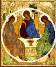THE PHYSICAL SENSE OF PLANCK CONSTANT IN MODEL OF "VACUUM CRYSTAL"
Planck constant as energy of unit mode of vacuum field. L.Regelson
Einstein relation for spectral density of radiation energy ρ in the conditions of thermal balance – is given by:

N
2  + B21 ρN2 = B12 ρN1                            (1)

Here B
12  = B21 = B – probabilities of transitions up and down under the influence of an electromagnetic field, A21 = A – probability of spontaneous transition from upper level on lower one.

Expression (1) can be written in the form:

N
2(ρ + A/B) = ρN1                                 (1a)

Taking account of Boltzman distributions of occupation numbers N1 and N2, and also of  Rayleigh-Jeans formula, the relation can be derived:

A/B =
8πһ/λ(3) = n(λ)һ                            (2)

where

n(λ) = 8π/λ(3)                                        (3)

– volume density of modes with wave length  λ.

Unlike an electromagnetic field which causes transitions both downwards and upwards,  the vacuum field is capable to cause only transitions  from upper level onto lower one.

Let's try to explain this "asymmetry" of action of a vacuum field by means of the model of "crystal vacuum” or “a world crystal”.

This excitation field, which causes spontaneous transitions, can be presented as analogue of phonon field in usual crystal. By assumption “acoustic phonons“ have place here, they can be identified with “longitudinal photons” in quantum electrodynamics which are responsible for Coulomb interaction. But Coulomb field is created by the ordered stream of longitudinal photons from a source whereas spontaneous transitions are caused by fluctuations of a chaotic field of longitudinal photons.

The model of "vacuum crystal” allows to explain the origin of energy which is necessary for spontaneous transition. The electronic cloud in atom is constantly influenced by a chaotic vacuum phonon field
ρvac. Casual phases coincidence of these phonons, creating a fluctuation of certain type, leads to disintegration (decay) of stationary state and to transition of  electronic cloud (under Coulomb potential action) in lower stationary state. Such transition is accompanied by emission electromagnetic wave train ("photon") which frequency is equal to a difference of frequencies of stationary conditions.

N
2 (ρ + ρvac) = ρN1                                 (5)

According to this expression, ρvac could be interpreted as volume spectral density of energy of the hypothetical vacuum field responsible for "spontaneous" transitions. As n(λ) according to (3), represents modes density, so Planck constant һ means energy of an individual mode of vacuum “phonon” fields.
The volume density of energy of this field in the range of frequencies ∆ν is:

∆ε = ρvac ν = ρvac ∆(c/λ)

Substitution of (4) and (3) into this expression gives:

dε/dλ = 8πһc/λ
(5)                                (6)

Full energy in volume unit (or the  pressure of field P) may be defined by integration on all possible wave lengths. If to denote a vacuum lattice constant as
a, the minimum wave length will be: λ0 = 2а. Disregarding reduction of modes density in comparison with (3)  when  λ → a, it is possible to receive the rough (obviously overrated) estimate of pressure, by means of integration (6) from λ0 to ∞:

P =
dε = 4πһс/а(4)                                (7)

Thus, volume energy density of  “phonon” vacuum field (it is the same as the field of "longitudinal photons”) is inverse proportional  4-th degrees of vacuum crystal  lattice constant

If our assumption is true: the lattice knots are formed by quarks – the lattice constant should be several times more than characteristic diameter of quark,
i.e.
а ~ 10(-18) m.

Substitution of this value in (7) gives an estimation of energy density:
P ~ 10
(31) J/m(3) = 10(31) N/m(2)

Energy of phonon vacuum field in atom volume [
ra ≈ 10(-10) m] thus will be Еa ~ 10 J.

Such a great scale of energy cannot serve as a refutation of the offered model: spontaneous transitions are caused not by field itself, but only by field fluctuations.